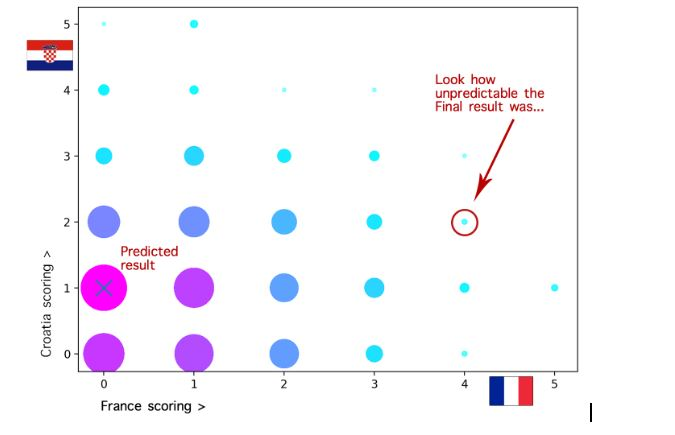A few days before the start of the 2018 FIFA World Cup in Russia, Globant’s Artificial Intelligence and Big Data studios decided to use proprietary technology to predict match results. We’ve already developed these technologies, so why not have a little fun with them?

We developed Globot AI, an Artificial Intelligence engine that used statistical simulation to predict the results of all 64 matches of the World Cup, based on the data of the players that make up each team. Let’s explore how Globot AI works

Each team’s data was generated using the FIFA 2018 game database, which is widely available online. This dataset covers 75 characteristics, such as acceleration, agility, ball control, dribbling, and penalties, of almost 18,000 players. These characteristics were used to calculate  different offensive and defensive scores for each of the 32 teams that played the World Cup.

Once we characterized the teams, we used statistical simulation techniques to obtain the result, for example, of a thousand matches between two given teams. The conditions of each match were simulated by slightly varying the characteristics of the teams in each simulated game, using normal distributions. Once we established the conditions of each game that would be simulated, we simulated the score of each team using Poisson’s distributions, which are optimal to describe the occurrence of certain events in certain periods of time, such as the goals of team A, since it plays against team B, in a football game.

As a result of this experiment, we obtained, for each match between team A and team B, a thousand possible scores that team A will score against team B, and vice versa, which also makes up the same number of possible results for the match. The most common result in that scenario was the result that the Globot AI put forward as a prediction (with its respective percentage over the total number of simulated matches).

For the final match between France and Croatia, Globot AI posted a 0-1 prediction, naming Croatia the World Cup winner. According to the simulation, France would end with a score of 0 goals in 392 of 1000 matches, and Croatia would end with a score of 1 goal in 378 of 1000 matches. Analyzing the results in more detail, we see the following graphic representation of the possible results for this match (the ‘x’ marks the most common result).The most interesting thing about this experiment is not the method used to simulate the matches or whether the predicted result for each match happened. Rather, it was learning what it means to make a statistical inference and how this kind of technique can even help us in the generation of synthetic datasets to feed the training of a certain algorithm.

According to Wikipedia says,

statistical inference is the process of using data analysis to deduce properties of an underlying probability distribution. Inferential statistical analysis infers properties of a population, for example by testing hypotheses and deriving estimates.

This concept differs from that of descriptive statistics, which is solely concerned with properties of the observed data.

The problem with statistical inference is that it is only mostly wrong (which can lead to a very deep discussion about philosophical interpretations of probability*). In a nutshell, any statistical inference requires several assumptions, or models. A statistical model is a set of assumptions about the generation of the observed data and similar data, so any limitation of the model is a problem in the inference (and we know that models are inherently less complex than the reality they are trying to explain). In our case, we generated observed data (results of matches) using models that simulate each game’s conditions and the probability distribution of the number of goals a team can make. We need to understand then the implications of statistical inference to know how to evaluate any prediction based on these techniques.

*If anyone wants to engage in a deeper this discussion, I recommend a lecture by the Computation & Cognition Lab of Stanford University, called Probabilistic reasoning and statistical inference.

Haldo Spontón

Tech Manager – AI & Big Data Studios @Globant